# Lorene::Time_slice Class Reference [Time evolution (under development)]

Spacelike time slice of a 3+1 spacetime. More...

#include <time_slice.h>

Inheritance diagram for Lorene::Time_slice: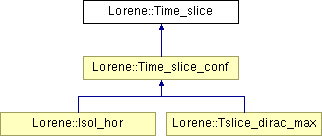List of all members.

## Public Member Functions

Time_slice (const Scalar &lapse_in, const Vector &shift_in, const Sym_tensor &gamma_in, const Sym_tensor &kk_in, int depth_in=3)
General constructor (Hamiltonian-like).
Time_slice (const Scalar &lapse_in, const Vector &shift_in, const Evolution_std< Sym_tensor > &gamma_in)
General constructor (Lagrangian-like).
Time_slice (const Map &mp, const Base_vect &triad, int depth_in=3)
Constructor as standard time slice of flat spacetime (Minkowski).
Time_slice (const Map &mp, const Base_vect &triad, FILE *fich, bool partial_read, int depth_in=3)
Constructor from binary file.
Time_slice (const Time_slice &)
Copy constructor.
virtual ~Time_slice ()
Destructor.
void operator= (const Time_slice &)
Assignment to another Time_slice.
void set_scheme_order (int ord)
Sets the order of the finite-differences scheme.
int get_scheme_order () const
Gets the order of the finite-differences scheme.
int get_latest_j () const
Gets the latest value of time step index.
const Evolution_std< double > & get_time () const
Gets the time coordinate t at successive time steps.
virtual const Scalarnn () const
Lapse function N at the current time step (jtime ).
virtual const Vectorbeta () const
shift vector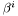at the current time step (jtime )
const Metricgam () const
Induced metric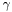at the current time step (jtime ).
virtual const Sym_tensorgam_dd () const
Induced metric (covariant components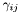) at the current time step (jtime ).
virtual const Sym_tensorgam_uu () const
Induced metric (contravariant components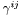) at the current time step (jtime ).
virtual const Sym_tensork_dd () const
Extrinsic curvature tensor (covariant components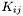) at the current time step (jtime ).
virtual const Sym_tensork_uu () const
Extrinsic curvature tensor (contravariant components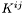) at the current time step (jtime ).
virtual const Scalartrk () const
Trace K of the extrinsic curvature at the current time step (jtime ).
Tbl check_hamiltonian_constraint (const Scalar *energy_density=0x0, ostream &ost=cout, bool verb=true) const
Checks the level at which the hamiltonian constraint is verified.
Tbl check_momentum_constraint (const Vector *momentum_density=0x0, ostream &ost=cout, bool verb=true) const
Checks the level at which the momentum constraints are verified.
Tbl check_dynamical_equations (const Sym_tensor *strain_tensor=0x0, const Scalar *energy_density=0x0, ostream &ost=cout, bool verb=true) const
Checks the level at which the dynamical equations are verified.
Returns the ADM mass (geometrical units) at the current step.
void save (const char *rootname) const
Saves in a binary file.

## Protected Member Functions

Time_slice (int depth_in)
Special constructor for derived classes.
virtual void del_deriv () const
Deletes all the derived quantities.
void set_der_0x0 () const
Sets to 0x0 all the pointers on derived quantities.
virtual ostream & operator>> (ostream &) const
Operator >> (virtual function called by the operator<<).
virtual void sauve (FILE *fich, bool partial_save) const
Total or partial saves in a binary file.

## Protected Attributes

int depth
Number of stored time slices.
int scheme_order
Order of the finite-differences scheme for the computation of time derivatives.
int jtime
Time step index of the latest slice.
Evolution_std< double > the_time
Time label of each slice.
Evolution_std< Sym_tensorgam_dd_evol
Values at successive time steps of the covariant components of the induced metric.
Evolution_std< Sym_tensorgam_uu_evol
Values at successive time steps of the contravariant components of the induced metric.
Evolution_std< Sym_tensork_dd_evol
Values at successive time steps of the covariant components of the extrinsic curvature tensor.
Evolution_std< Sym_tensork_uu_evol
Values at successive time steps of the contravariant components of the extrinsic curvature tensor.
Evolution_std< Scalarn_evol
Values at successive time steps of the lapse function N.
Evolution_std< Vectorbeta_evol
Values at successive time steps of the shift vector.
Evolution_std< Scalartrk_evol
Values at successive time steps of the trace K of the extrinsic curvature.
ADM mass at each time step, since the creation of the slice.
Metricp_gamma
Pointer on the induced metric at the current time step (jtime).

## Friends

ostream & operator<< (ostream &, const Time_slice &)
Display.

## Detailed Description

Spacelike time slice of a 3+1 spacetime.

()

Definition at line 176 of file time_slice.h.

## Constructor & Destructor Documentation

 Lorene::Time_slice::Time_slice ( const Scalar & lapse_in, const Vector & shift_in, const Sym_tensor & gamma_in, const Sym_tensor & kk_in, int depth_in = 3 )

General constructor (Hamiltonian-like).

Parameters:
 lapse_in lapse function N shift_in shift vector gamma_in induced metric (covariant or contravariant components) kk_in extrinsic curvature (covariant or contravariant components) depth_in number of stored time slices; this parameter is used to set the scheme_order member with scheme_order = depth_in - 1. scheme_order can be changed afterwards by the method set_scheme_order(int).

Definition at line 115 of file time_slice.C.

 Lorene::Time_slice::Time_slice ( const Scalar & lapse_in, const Vector & shift_in, const Evolution_std< Sym_tensor > & gamma_in )

General constructor (Lagrangian-like).

Parameters:
 lapse_in lapse function N shift_in shift vector gamma_in induced metric (covariant or contravariant components) at various time steps; note that the scheme_order member is set to gamma_in.size - 1. scheme_order can be changed afterwards by the method set_scheme_order(int).

Definition at line 158 of file time_slice.C.

References set_der_0x0().

 Lorene::Time_slice::Time_slice ( const Map & mp, const Base_vect & triad, int depth_in = 3 )

Constructor as standard time slice of flat spacetime (Minkowski).

Parameters:
 mp Mapping on which the various Lorene fields will be constructed triad vector basis with respect to which the various tensor components will be defined depth_in number of stored time slices; this parameter is used to set the scheme_order member with scheme_order = depth_in - 1. scheme_order can be changed afterwards by the method set_scheme_order(int).

Definition at line 183 of file time_slice.C.

 Lorene::Time_slice::Time_slice ( const Map & mp, const Base_vect & triad, FILE * fich, bool partial_read, int depth_in = 3 )

Constructor from binary file.

The binary file must have been created by method save.

Parameters:
 mp Mapping on which the various Lorene fields will be constructed triad vector basis with respect to which the various tensor components will be defined fich file containing the saved Time_slice partial_read indicates whether the full object must be read in file or whether the final construction is devoted to a constructor of a derived class depth_in number of stored time slices; the given value must coincide with that stored in the file.

Definition at line 238 of file time_slice.C.

 Lorene::Time_slice::Time_slice ( const Time_slice & tin )

Copy constructor.

Definition at line 317 of file time_slice.C.

References set_der_0x0().

 Lorene::Time_slice::Time_slice ( int depth_in )  [explicit, protected]

Special constructor for derived classes.

Definition at line 336 of file time_slice.C.

References set_der_0x0().

 Lorene::Time_slice::~Time_slice ( )  [virtual]

Destructor.

Definition at line 360 of file time_slice.C.

References del_deriv().

## Member Function Documentation

 double Lorene::Time_slice::adm_mass ( ) const [virtual]

Returns the ADM mass (geometrical units) at the current step.

Moreover this method updates adm_mass_evol if necessary.

Reimplemented in Lorene::Time_slice_conf, and Lorene::Tslice_dirac_max.

Definition at line 77 of file tslice_adm_mass.C.

 const Vector & Lorene::Time_slice::beta ( ) const [virtual]

shift vectorat the current time step (jtime )

Definition at line 90 of file time_slice_access.C.

References beta_evol, Lorene::Evolution< TyT >::is_known(), and jtime.

 Tbl Lorene::Time_slice::check_dynamical_equations ( const Sym_tensor * strain_tensor = 0x0, const Scalar * energy_density = 0x0, ostream & ost = cout, bool verb = true ) const

Checks the level at which the dynamical equations are verified.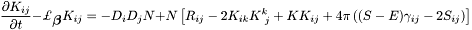Parameters:
 strain_tensor : a pointer on the strain_tensor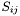measured by the Eulerian observer of 4-velocity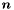; if this is the null pointer, it is assumed that= 0 (vacuum). energy_density : a pointer on the energy density E (see check_hamiltonian_constraint) ost : output stream for a formatted output of the result
Returns:
Tbl 3D of size the number of domains times 3 times 3 (corresponding to the rank-2 tensor, with the symmetry in the components) containing the absolute ( if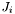= 0 ) or the relative (in presence of matter) error in max version.

Definition at line 142 of file tslice_check_einstein.C.

 Tbl Lorene::Time_slice::check_hamiltonian_constraint ( const Scalar * energy_density = 0x0, ostream & ost = cout, bool verb = true ) const

Checks the level at which the hamiltonian constraint is verified.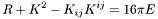Parameters:
 energy_density : a pointer on the energy density E measured by the Eulerian observer of 4-velocity; if this is the null pointer, it is assumed that E = 0 (vacuum). ost : output stream for a formatted output of the result
Returns:
Tbl of size the number of domains containing the absolute ( if E = 0 ) or the relative (in presence of matter) error in max version.

Definition at line 82 of file tslice_check_einstein.C.

 Tbl Lorene::Time_slice::check_momentum_constraint ( const Vector * momentum_density = 0x0, ostream & ost = cout, bool verb = true ) const

Checks the level at which the momentum constraints are verified.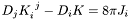Parameters:
 momentum_density : a pointer on the momentum densitymeasured by the Eulerian observer of 4-velocity; if this is the null pointer, it is assumed that= 0 (vacuum). ost : output stream for a formatted output of the result
Returns:
Tbl 2D of size the number of domains times 3 (components)containing the absolute ( if= 0 ) or the relative (in presence of matter) error in max version.

Definition at line 112 of file tslice_check_einstein.C.

 void Lorene::Time_slice::del_deriv ( ) const [protected, virtual]

Deletes all the derived quantities.

Reimplemented in Lorene::Time_slice_conf.

Definition at line 370 of file time_slice.C.

References adm_mass_evol, Lorene::Evolution< TyT >::downdate(), jtime, p_gamma, and set_der_0x0().

 const Metric & Lorene::Time_slice::gam ( ) const

Induced metricat the current time step (jtime ).

Definition at line 98 of file time_slice_access.C.

References gam_dd(), and p_gamma.

 const Sym_tensor & Lorene::Time_slice::gam_dd ( ) const [virtual]

Induced metric (covariant components) at the current time step (jtime ).

Reimplemented in Lorene::Time_slice_conf.

Definition at line 110 of file time_slice_access.C.

 const Sym_tensor & Lorene::Time_slice::gam_uu ( ) const [virtual]

Induced metric (contravariant components) at the current time step (jtime ).

Reimplemented in Lorene::Time_slice_conf.

Definition at line 125 of file time_slice_access.C.

 int Lorene::Time_slice::get_latest_j ( ) const [inline]

Gets the latest value of time step index.

Definition at line 346 of file time_slice.h.

References jtime.

 int Lorene::Time_slice::get_scheme_order ( ) const [inline]

Gets the order of the finite-differences scheme.

Definition at line 343 of file time_slice.h.

References scheme_order.

 const Evolution_std& Lorene::Time_slice::get_time ( ) const [inline]

Gets the time coordinate t at successive time steps.

Definition at line 349 of file time_slice.h.

References the_time.

 const Sym_tensor & Lorene::Time_slice::k_dd ( ) const [virtual]

Extrinsic curvature tensor (covariant components) at the current time step (jtime ).

Reimplemented in Lorene::Time_slice_conf.

Definition at line 138 of file time_slice_access.C.

 const Sym_tensor & Lorene::Time_slice::k_uu ( ) const [virtual]

Extrinsic curvature tensor (contravariant components) at the current time step (jtime ).

Reimplemented in Lorene::Time_slice_conf.

Definition at line 160 of file time_slice_access.C.

 const Scalar & Lorene::Time_slice::nn ( ) const [virtual]

Lapse function N at the current time step (jtime ).

Reimplemented in Lorene::Time_slice_conf.

Definition at line 82 of file time_slice_access.C.

References Lorene::Evolution< TyT >::is_known(), jtime, and n_evol.

 void Lorene::Time_slice::operator= ( const Time_slice & tin )

Assignment to another Time_slice.

Reimplemented in Lorene::Isol_hor, Lorene::Time_slice_conf, and Lorene::Tslice_dirac_max.

Definition at line 391 of file time_slice.C.

References beta_evol, del_deriv(), depth, gam_dd_evol, gam_uu_evol, jtime, k_dd_evol, k_uu_evol, n_evol, scheme_order, the_time, and trk_evol.

 ostream & Lorene::Time_slice::operator>> ( ostream & flux ) const [protected, virtual]

Operator >> (virtual function called by the operator<<).

Reimplemented in Lorene::Isol_hor, Lorene::Time_slice_conf, and Lorene::Tslice_dirac_max.

Definition at line 414 of file time_slice.C.

 void Lorene::Time_slice::sauve ( FILE * fich, bool partial_save ) const [protected, virtual]

Total or partial saves in a binary file.

This protected method is to be called either from public method save or from method sauve of a derived class.

Parameters:
 fich binary file partial_save indicates whether the whole object must be saved.

Reimplemented in Lorene::Isol_hor, Lorene::Time_slice_conf, and Lorene::Tslice_dirac_max.

Definition at line 510 of file time_slice.C.

 void Lorene::Time_slice::save ( const char * rootname ) const

Saves in a binary file.

The saved data is sufficient to restore the whole time slice via the constructor from file.

Parameters:
 rootname root for the file name; the current time step index will be appended to it.

Definition at line 464 of file time_slice.C.

 void Lorene::Time_slice::set_der_0x0 ( ) const [protected]

Sets to 0x0 all the pointers on derived quantities.

Reimplemented in Lorene::Time_slice_conf.

Definition at line 380 of file time_slice.C.

References p_gamma.

 void Lorene::Time_slice::set_scheme_order ( int ord )  [inline]

Sets the order of the finite-differences scheme.

Definition at line 334 of file time_slice.h.

References scheme_order.

 const Scalar & Lorene::Time_slice::trk ( ) const [virtual]

Trace K of the extrinsic curvature at the current time step (jtime ).

Reimplemented in Lorene::Time_slice_conf, and Lorene::Tslice_dirac_max.

Definition at line 180 of file time_slice_access.C.

## Friends And Related Function Documentation

 ostream& operator<< ( ostream & , const Time_slice & )  [friend]

Display.

## Member Data Documentation

 Evolution_full Lorene::Time_slice::adm_mass_evol [mutable, protected]

ADM mass at each time step, since the creation of the slice.

At a given time step j, adm_mass_evol[j] is a 1-D Tbl of size the number nz of domains, containing the "ADM mass" evaluated at the outer boundary of each domain. The true ADM mass is thus the last value, i.e. adm_mass_evol[j](nz-1).

Definition at line 236 of file time_slice.h.

 Evolution_std Lorene::Time_slice::beta_evol [mutable, protected]

Values at successive time steps of the shift vector.

Definition at line 222 of file time_slice.h.

 int Lorene::Time_slice::depth [protected]

Number of stored time slices.

Definition at line 182 of file time_slice.h.

 Evolution_std Lorene::Time_slice::gam_dd_evol [mutable, protected]

Values at successive time steps of the covariant components of the induced metric.

Definition at line 201 of file time_slice.h.

 Evolution_std Lorene::Time_slice::gam_uu_evol [mutable, protected]

Values at successive time steps of the contravariant components of the induced metric.

Definition at line 206 of file time_slice.h.

 int Lorene::Time_slice::jtime [protected]

Time step index of the latest slice.

Definition at line 193 of file time_slice.h.

 Evolution_std Lorene::Time_slice::k_dd_evol [mutable, protected]

Values at successive time steps of the covariant components of the extrinsic curvature tensor.

Definition at line 211 of file time_slice.h.

 Evolution_std Lorene::Time_slice::k_uu_evol [mutable, protected]

Values at successive time steps of the contravariant components of the extrinsic curvature tensor.

Definition at line 216 of file time_slice.h.

 Evolution_std Lorene::Time_slice::n_evol [mutable, protected]

Values at successive time steps of the lapse function N.

Definition at line 219 of file time_slice.h.

 Metric* Lorene::Time_slice::p_gamma [mutable, protected]

Pointer on the induced metric at the current time step (jtime).

Definition at line 242 of file time_slice.h.

 int Lorene::Time_slice::scheme_order [protected]

Order of the finite-differences scheme for the computation of time derivatives.

This order is not constant and can be adjusted via set_scheme_order() .

Definition at line 190 of file time_slice.h.

 Evolution_std Lorene::Time_slice::the_time [protected]

Time label of each slice.

Definition at line 196 of file time_slice.h.

 Evolution_std Lorene::Time_slice::trk_evol [mutable, protected]

Values at successive time steps of the trace K of the extrinsic curvature.

Definition at line 227 of file time_slice.h.

The documentation for this class was generated from the following files:

Generated on 7 Dec 2019 for LORENE by1.6.1